official practice test 3 section 4 q.no.35 how to solve?

The question tells you that the store’s average after 10 ratings is 75. That means that the first 10 ratings have a sum of 750, because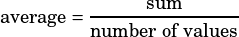,. In this case,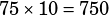.

You need the first 20 ratings to have an average of 85, which means you need the sum of the first 20 ratings to be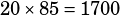. So far so good?

Now, we know that the first 10 ratings added to 750, so we need the next 10 ratings to add to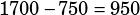.

Since we want to know the smallest possible value for the 11th rating, let’s assume that the 12th-20th ratings are the highest they can be, 100. In other words, let’s assume there are 9 perfect 100 ratings, and set the 11th rating equal to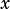.